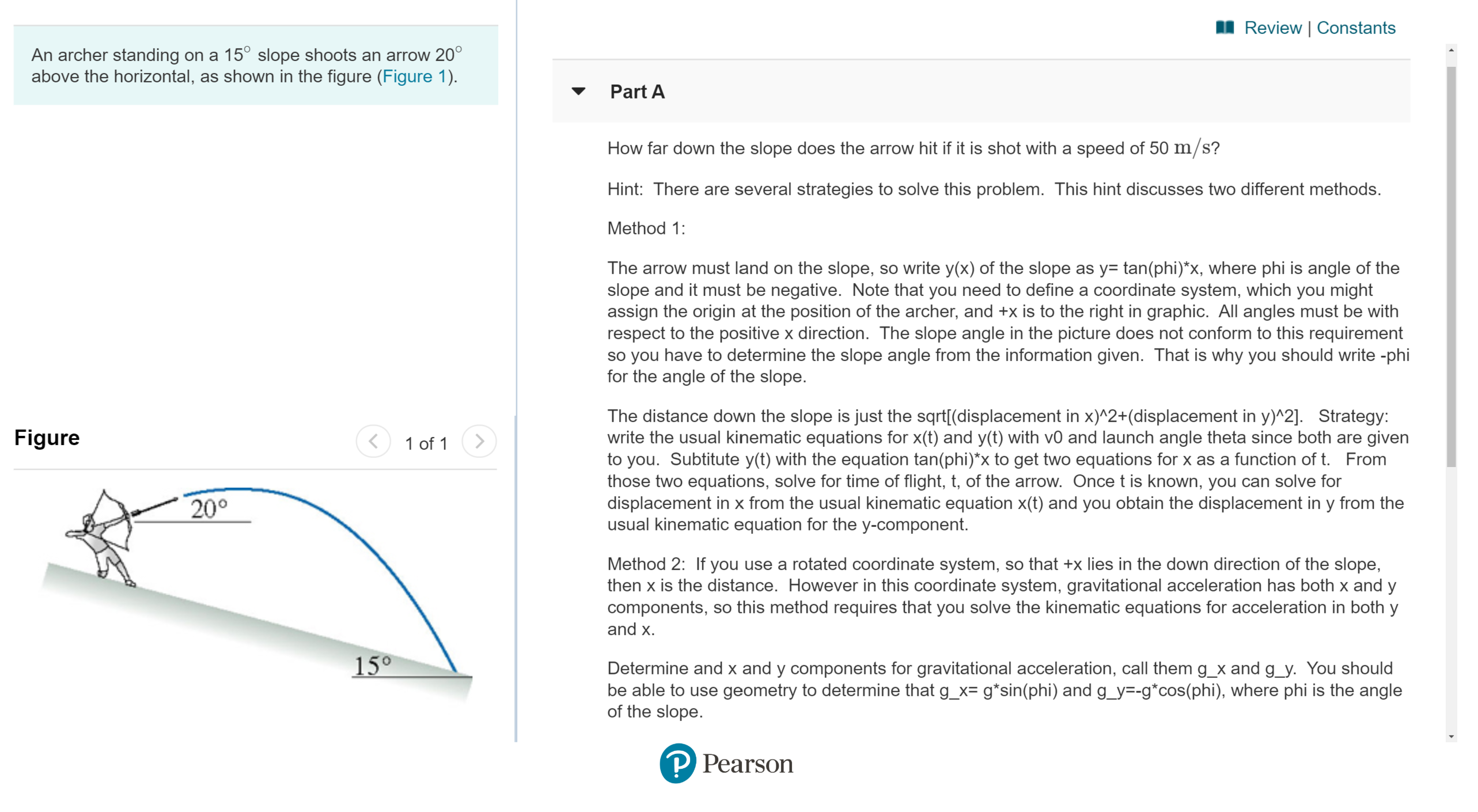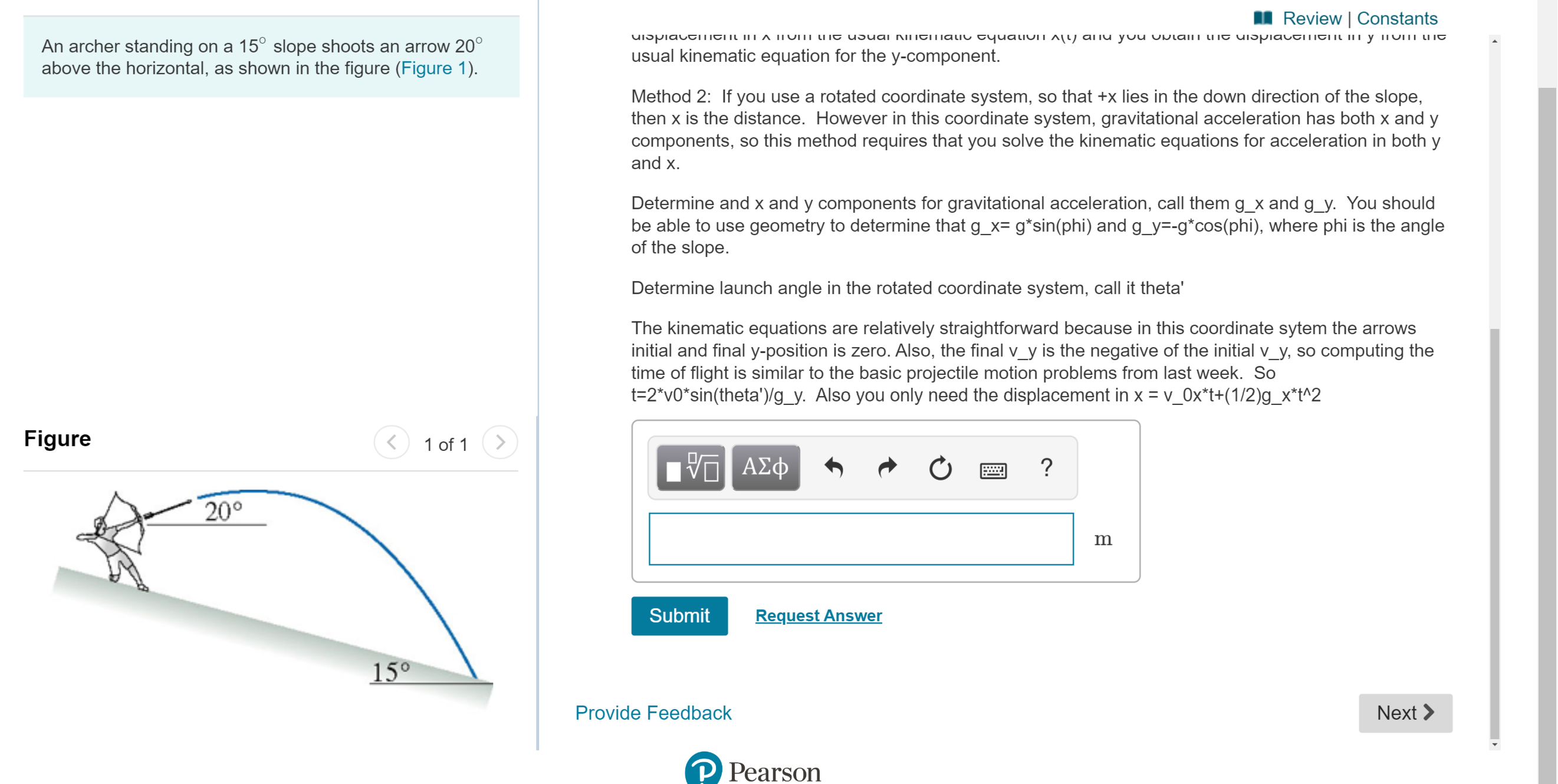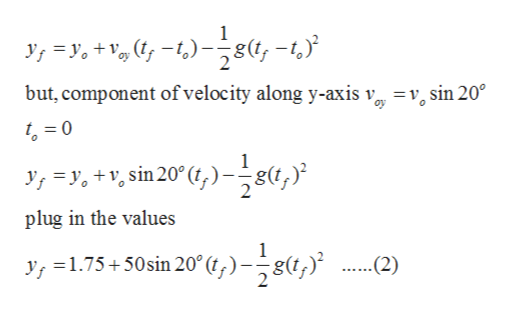# Review | ConstantsAn archer standing on a 15° slope shoots an arrow 20°above the horizontal, as shown in the figure (Figure 1)Part AHow far down the slope does the arrow hit if it is shot with a speed of 50 m/s?Hint: There are several strategies to solve this problem. This hint discusses two different methods.Method 1:The arrow must land on the slope, so write y(x) of the slope as y= tan(phi)*x, where phi is angle of theslope and it must be negative. Note that you need to define a coordinate system, which you mightassign the origin at the position of the archer, and +x is to the right in graphic. All angles must be withrespect to the positive x direction. The slope angle in the picture does not conform to this requirementso you have to determine the slope angle from the information given. That is why you should write-phifor the angle of the slope.The distance down the slope is just the sqrt[(displacement in x)^2+(displacement in y)^2]. Strategy:write the usual kinematic equations for x(t) and y(t) with v0 and launch angle theta since both are givento you. Subtitute y(t) with the equation tan(phi)*x to get two equations for x as a function of t. Fromthose two equations, solve for time of flight, t, of the arrow. Once t is known, you can solve fordisplacement in x from the usual kinematic equation x(t) and you obtain the displacement in y from theusual kinematic equation for the y-component.Figure1 of 120°Method 2: If you use a rotated coordinate system, so that +x lies in the down direction of the slope,then x is the distance. However in this coordinate system, gravitational acceleration has both x and ycomponents, so this method requires that you solve the kinematic equations for acceleration in both yand x15°Determine and x and y components for gravitational acceleration, call them g_x and g_y. You shouldbe able to use geometry to determine that g_x= g*sin(phi) and g_y=-g*cos(phi), where phi is the angleof the slopeP Pearson Review | ConstantsuiSpiactITEN TA IIUMI UIE usuai NUEITIALIU EquaLIUIT A[LY aiu yuu UDlaii iE ulSpiauEITIENN ILI y inUTI LUIEAn archer standing on a 15° slope shoots an arrow 20°above the horizontal, as shown in the figure (Figure 1)usual kinematic equation for the y-component.Method 2: If you use a rotated coordinate system, so that +x lies in the down direction of the slope,then x is the distance. However in this coordinate system, gravitational acceleration has both x and ycomponents, so this method requires that you solve the kinematic equations for acceleration in both yand xDetermine and x and y components for gravitational acceleration, call them g_x and g_y. You shouldbe able to use geometry to determine that g_x= g*sin(phi) and g_y=-g*cos(phi), where phi is the angleof the slopeDetermine launch angle in the rotated coordinate system, call it theta'The kinematic equations are relatively straightforward because in this coordinate sytem the arrowsinitial and final y-position is zero. Also, the final v_y is the negative of the initial v_y, so computing thetime of flight is similar to the basic projectile motion problems from last week. Sot-2"v0*sin(theta')/g_y. Also you only need the displacement in x v_Ox*t+(1/2)g_x*t^2Figure1 of 1V ΑΣφ20°mRequest AnswerSubmit15°Provide FeedbackNextPearson

Question
10 viewshelp_outlineImage TranscriptioncloseReview | Constants An archer standing on a 15° slope shoots an arrow 20° above the horizontal, as shown in the figure (Figure 1) Part A How far down the slope does the arrow hit if it is shot with a speed of 50 m/s? Hint: There are several strategies to solve this problem. This hint discusses two different methods. Method 1: The arrow must land on the slope, so write y(x) of the slope as y= tan(phi)*x, where phi is angle of the slope and it must be negative. Note that you need to define a coordinate system, which you might assign the origin at the position of the archer, and +x is to the right in graphic. All angles must be with respect to the positive x direction. The slope angle in the picture does not conform to this requirement so you have to determine the slope angle from the information given. That is why you should write-phi for the angle of the slope. The distance down the slope is just the sqrt[(displacement in x)^2+(displacement in y)^2]. Strategy: write the usual kinematic equations for x(t) and y(t) with v0 and launch angle theta since both are given to you. Subtitute y(t) with the equation tan(phi)*x to get two equations for x as a function of t. From those two equations, solve for time of flight, t, of the arrow. Once t is known, you can solve for displacement in x from the usual kinematic equation x(t) and you obtain the displacement in y from the usual kinematic equation for the y-component. Figure 1 of 1 20° Method 2: If you use a rotated coordinate system, so that +x lies in the down direction of the slope, then x is the distance. However in this coordinate system, gravitational acceleration has both x and y components, so this method requires that you solve the kinematic equations for acceleration in both y and x 15° Determine and x and y components for gravitational acceleration, call them g_x and g_y. You should be able to use geometry to determine that g_x= g*sin(phi) and g_y=-g*cos(phi), where phi is the angle of the slope P Pearson fullscreenhelp_outlineImage TranscriptioncloseReview | Constants uiSpiactITEN TA IIUMI UIE usuai NUEITIALIU EquaLIUIT A[LY aiu yuu UDlaii iE ulSpiauEITIENN ILI y inUTI LUIE An archer standing on a 15° slope shoots an arrow 20° above the horizontal, as shown in the figure (Figure 1) usual kinematic equation for the y-component. Method 2: If you use a rotated coordinate system, so that +x lies in the down direction of the slope, then x is the distance. However in this coordinate system, gravitational acceleration has both x and y components, so this method requires that you solve the kinematic equations for acceleration in both y and x Determine and x and y components for gravitational acceleration, call them g_x and g_y. You should be able to use geometry to determine that g_x= g*sin(phi) and g_y=-g*cos(phi), where phi is the angle of the slope Determine launch angle in the rotated coordinate system, call it theta' The kinematic equations are relatively straightforward because in this coordinate sytem the arrows initial and final y-position is zero. Also, the final v_y is the negative of the initial v_y, so computing the time of flight is similar to the basic projectile motion problems from last week. So t-2"v0*sin(theta')/g_y. Also you only need the displacement in x v_Ox*t+(1/2)g_x*t^2 Figure 1 of 1 V ΑΣφ 20° m Request Answer Submit 15° Provide Feedback Next Pearson fullscreen
check_circle

Step 1

Given:

Angle of slope = 15o

Angle at which arrow shoot = 20o

Let, average height of the man, yo = 1.75 m

Initial speed of the arrow = 50 m/s

Step 2

Slope on which arrow land can be calculated as

Step 3

Final vertical positio...help_outlineImage Transcriptionclose,y. +v G, -t,);8&, -t,) but, component of velocity along y-axis v v sin 20 0 J,y +v,sin 20° )8, plug in the values y, 1.75+50sin 20 ,) 8, 2) fullscreen

### Want to see the full answer?

See Solution

#### Want to see this answer and more?

Solutions are written by subject experts who are available 24/7. Questions are typically answered within 1 hour.*

See Solution
*Response times may vary by subject and question.
Tagged in

### Kinematics